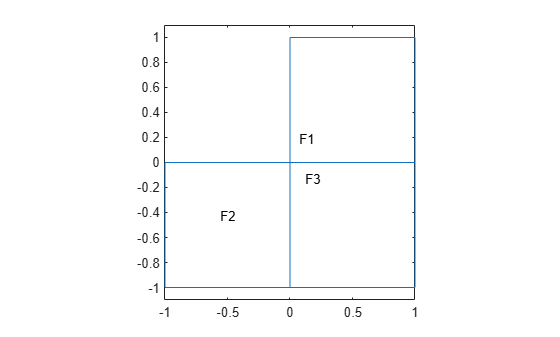## View, Edit, and Delete PDE Coefficients

### View Coefficients

A PDE model stores coefficients in its `EquationCoefficients` property. Suppose `model` is the name of your model. Obtain the coefficients:

`coeffs = model.EquationCoefficients;`

To see the active coefficient assignment for a region, call the `findCoefficients` function. For example, create a model and view the geometry.

```model = createpde(); geometryFromEdges(model,@lshapeg); pdegplot(model,"FaceLabels","on") ylim([-1.1,1.1]) axis equal```Specify constant coefficients over all the regions in the model.

`specifyCoefficients(model,"m",0,"d",0,"c",1,"a",0,"f",2);`

Specify a different `f` coefficient on each subregion.

```specifyCoefficients(model,"m",0,"d",0,"c",1,"a",0,"f",3,"Face",2); specifyCoefficients(model,"m",0,"d",0,"c",1,"a",0,"f",4,"Face",3);```

Change the specification to have nonzero `a` on region 2.

`specifyCoefficients(model,"m",0,"d",0,"c",1,"a",1,"f",3,"Face",2);`

View the coefficient assignment for region 2.

```coeffs = model.EquationCoefficients; findCoefficients(coeffs,"Face",2)```
```ans = CoefficientAssignment with properties: RegionType: 'face' RegionID: 2 m: 0 d: 0 c: 1 a: 1 f: 3 ```

This shows the "last assignment wins" characteristic.

View the coefficient assignment for region 1.

`findCoefficients(coeffs,"Face",1)`
```ans = CoefficientAssignment with properties: RegionType: 'face' RegionID: [1 2 3] m: 0 d: 0 c: 1 a: 0 f: 2 ```

The active coefficient assignment for region 1 includes all three regions, though this assignment is no longer active for regions 2 and 3.

### Delete Existing Coefficients

To delete all the coefficients in your PDE model, use `delete`. Suppose `model` is the name of your model. Remove all coefficients from `model`.

`delete(model.EquationCoefficients)`

To delete specific coefficient assignments, delete them from the `model.EquationCoefficients.CoefficientAssignments` vector.

```coefv = model.EquationCoefficients.CoefficientAssignments; delete(coefv(2))```

Tip

You do not need to delete coefficients; you can override them by calling `specifyCoefficients` again. However, deleting unused assignments can make your model smaller.

### Change a Coefficient Assignment

To change a coefficient assignment, you need the coefficient handle. To get the coefficient handle:

• Retain the handle when using `specifyCoefficients`. For example,

`coefh1 = specifyCoefficients(model,"m",m,"d",d,"c",c,"a",a,"f",f);`
• Obtain the handle using `findCoefficients`. For example,

```coeffs = model.EquationCoefficients; coefh1 = findCoefficients(coeffs,"Face",2);```

You can change any property of the coefficient handle. For example,

```coefh1.RegionID = [1,3]; coefh1.a = 2; coefh1.c = @ccoeffun;```

Note

Editing an existing assignment in this way does not change its priority. For example, if the active coefficient in region 3 was assigned after `coefh1`, then editing `coefh1` to include region 3 does not make `coefh1` the active coefficient in region 3.